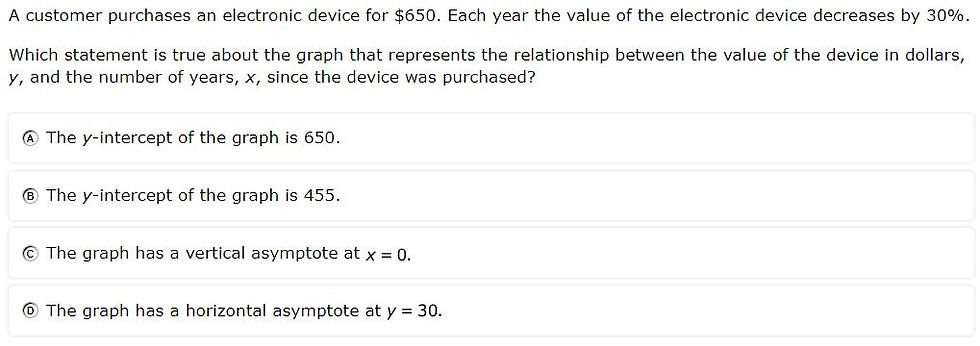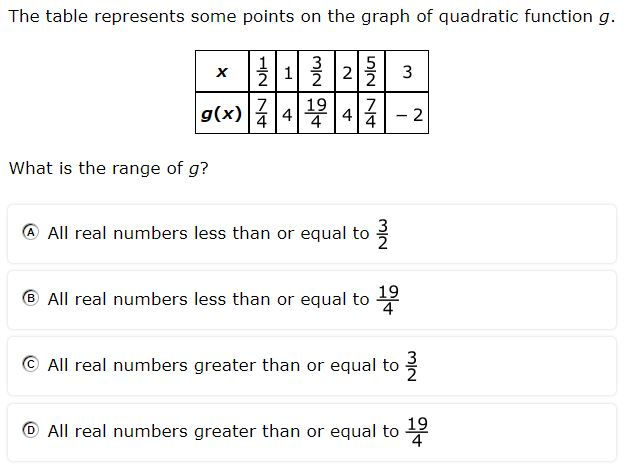top of page
Search

# Lessons learned from the 2023 Algebra 1 STAAR

The 2023 Algebra 1 STAAR introduced statewide online testing and several new item types. Using a modified version of the statewide item analysis report, I examined the readiness standards that had less than 50% mastery. Each standard has both an analysis of the items themselves to infer what made them so difficult and instructional implications for educators to ensure a more successful 2024 STAAR test.

 Standard # of items % mastery A.11B 2 21 A.7C 1 30 A.8A 1 30 A.2A 2 33.5 A.9D 2 34.5 A.2I 1 39 A.5A 2 42.5 A.9C 2 43.5 A.6A 2 45.5 A.9C 2 49.5

Access the slide deck here.

### A.11B - 21% overall mastery

simplify numeric and algebraic expressions using the laws of exponents, including integral and rational exponents

#10 - 38% correct#35 - 4% full credit, 38% partial credit, 58% no credit###### Analysis
• Fractions add a layer of complexity to dividing exponents or finding the power of a power

• The drag and drop response required you to use the same number twice (not all drag and drops allow using a response more than once)

###### Instructional Implications
• Utilize fractions in when asking students to multiply or divide by exponents

• Bundle multiple laws of exponents into a single problem (e.g., radicals and division)

• Have students practice rewriting dividing exponents as multiplying by negative exponents (e.g., x^4/x^2 = x^4 (x^-2) )

### A.7C - 30% overall mastery

determine the effects on the graph of the parent function f(x) = x2 when f(x) is replaced by af(x), f(x) + d, f(x - c), f(bx) for specific values of a, b, c, and d

#38 - 30% full credit, 35% partial credit, 35% no credit###### Analysis
• Drag and drop removed certainty for partial guesses

• Students had to analyze both a vertical and horizontal transformation simultaneously

• The horizontal transformation (- 3) actually moves the vertex to the right

###### Instructional Implications
• Bundle problems so that students multiple transformations together

• Have students practice graphing the child function (correctly with exponent) to analyze the vertex

Watch the full walkthrough of all 50 items on the 2023 Algebra 1 STAAR below.

A.8A - 30% overall mastery

solve quadratic equations having real solutions by factoring, taking square roots, completing the square, and applying the quadratic formula

#41 - 30% correct###### Analysis
• Distribution of answer selections (30%, 28%, 27%, 14%) indicative of guessing

• Multiple strategies could have been used to solve the problem

###### Instructional Implications
• Focus on correctly applying quadratic formula

• Emphasize problems that require simplifying a radical

• Have students solve the same problem using at least two different strategies

### A.2A - 33.5% overall mastery

determine the domain and range of a linear function in mathematical problems; determine reasonable domain and range values for real-world situations, both continuous and discrete; and represent domain and range using inequalities

#7 - 33% full credit, 22% partial credit, 44% no credit#44 - 34% correct###### Analysis
• Responses were required in verbal form (rather than as inequalities)

• Students had to differentiate between open and closed circles

• Problems required analysis of both domain and range simultaneously

###### Instructional Implications
• Bundle problems so that students assess both domain and range together

• Have students draw rays that satisfy requirements of both domain and range

• Provide error analysis opportunities for students to disprove why graphs do NOT represent a given domain and range

### A.9D - 34.5% overall mastery

graph exponential functions that model growth and decay and identify key features, including y- intercept and asymptote, in mathematical and real-world problems

#9 - 14% full credit, 59% partial credit, 27% no credit#42 - 55% correct###### Analysis
• Both problems asked students to identify features of exponential graphs (i.e., asymptote, y-intercept)

• Common error for asymptote was most likely labeling x-axis as x = 0

###### Instructional Implications
• When working with standard form (y = ab ), also have students identify a as the y-intercept and explain why

• When given an exponential function in equation or graph form, have students create a real-world situation to describe it, including naming the y-intercept

### A.2I - 39% overall mastery

write systems of two linear equations given a table of values, a graph, and a verbal description

#37 - 39% correct###### Analysis
• Distribution of answer selections (16%, 21%, 24%, 39%) indicative of guessing

• Solving the problem required

• Finding slope of each line

• Substituting an ordered pair and solving for b for each line

• Transforming each resulting slope-intercept form equation into standard form

###### Instructional Implications
• Focus on applying correct sequence of formulas

• Practice building stamina and how to check their answers with substitution or by graphing discrete points along with the systems of equations

### A.5A - 42.5% overall mastery

solve linear equations in one variable, including those for which the application of the distributive property is necessary and for which variables are included on both sides

#4 - 22% correct#29 - 53% correct###### Analysis
• Distribution of answer selections for #4 (27%, 21%, 30%, 22%) indicative of guessing

• This type of problem (linear equations and distributive property) has never been asked without explicitly providing the equation

###### Instructional Implications
• Find and utilize verbal descriptions of linear equations (using the distributive property) that do not explicitly provide the equation

• Have students work in pairs to create their own problems (w/o explicit equations) and exchange with peers to solve

### A.9C - 43.5% overall mastery

write exponential functions in the form f(x) = ab^x (where b is a rational number) to describe problems arising from mathematical and real-world situations, including growth and decay

#14 - 37% correct#36 - 50% correct###### Analysis
• Students were not provided a growth factor for one problem

• Growth factor of 4% had to be translated to 1.04

###### Instructional Implications
• Have students practice finding growth/decay factor when given initial and first-year amount

• Provide rationale for translating a growth factor to 1 + decimal and a decay factor to 1 - decimal

• Give students percentage (e.g., decay rate of 22%) and practice converting to decay factor (e.g., 0.78)

### A.6A - 45.5% overall mastery

determine the domain and range of quadratic functions and represent the domain and range using inequalities

#11 - 47% correct#32 - 44% correct###### Analysis
• Students needed to graph at least one problem to correctly identify range

• Improper fractions in the table made visual sequencing challenging

###### Instructional Implications
• Reinforce difference between domain and range and that domain is rarely limited in quadratic equations

• Practice identifying range from a table by sketching a rough parabola with given points

• Practice plotting discrete points in a graphing calculator to visually determine range

### A.5C - 49.5% overall mastery

solve systems of two linear equations with two variables for mathematical and real-world problems

#23 - 68% correct#40 - 31% correct###### Analysis
• Distribution of answer selections for #40 (16%, 26%, 27%, 31%) indicative of guessing

• A system of equations question has never been asked using a table

###### Instructional Implications
• Have students practice taking systems of equations questions (verbal descriptions and equations) and translating them to tables

• Reinforce the qualities that define a system of equations (two or more equations that share variables) to better identify them in real-world situations